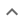•# Factoring multivariable polynomials

We think you wrote:

###This solution deals with factoring multivariable polynomials.

Solution found2*(x+3y)^2

## Step by Step Solution## Step  1  :

#### Equation at the end of step  1  :

  ((2 • (x2)) +  12xy) +  (2•32y2)
Step  2  :

#### Equation at the end of step  2  :

  (2x2 +  12xy) +  (2•32y2)


## Step  4  :

#### Pulling out like terms :

Pull out like factors :

2x2 + 12xy + 18y2  =   2 • (x2 + 6xy + 9y2)

#### Trying to factor a multi variable polynomial :

Factoring    x2 + 6xy + 9y2

Try to factor this multi-variable trinomial using trial and error

Found a factorization  :  (x + 3y)•(x + 3y)

#### Detecting a perfect square :

x2  +6xy  +9y2  is a perfect square

It factors into  (x+3y)•(x+3y)
which is another way of writing  (x+3y)2

How to recognize a perfect square trinomial:

• It has three terms

• Two of its terms are perfect squares themselves

• The remaining term is twice the product of the square roots of the other two terms

## Final result :

  2 • (x + 3y)2